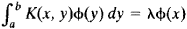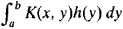# Eigenfunction

(redirected from Eigenfunctions)
Also found in: Dictionary, Medical.

## Eigenfunction

One of the solutions of an eigenvalue equation. A parameter-dependent equation that possesses nonvanishing solutions only for particular values (eigenvalues) of the parameter is an eigenvalue equation, the associated solutions being the eigenfunctions (sometimes eigenvectors). In older usage the terms characteristic equation and characteristic values (functions) are common. Eigenvalue equations appear in many contexts, including the solution of systems of linear algebraic equations (matrix equations), differential or partial differential equations, and integral equations. The importance of eigenfunctions and eigenvalues in applied mathematics results from the widespread applicability of linear equations as exact or approximate descriptions of physical systems. However, the most fundamental application of these concepts is in quantum mechanics where they enter into the definition and physical interpretation of the theory. Only linear eigenvalue equations will be discussed. See Eigenvalue (quantum mechanics), Energy level (quantum mechanics), Quantum mechanics

McGraw-Hill Concise Encyclopedia of Physics. © 2002 by The McGraw-Hill Companies, Inc.
The following article is from The Great Soviet Encyclopedia (1979). It might be outdated or ideologically biased.

## Eigenfunction

a concept of mathematical analysis. The solution of many problems in mathematical physics—for example, in the theory of vibrations and the theory of heat conduction—requires the finding of nontrivial solutions of homogeneous linear differential equations L(y) = Xy where the solutions satisfy particular boundary conditions. Such solutions are called ei-genfunctions of the problem, and the corresponding values of λ are called eigenvalues.

If a differential equation with appropriate boundary conditions is self-adjoint, then its eigenvalues are real, and the eigenfunctions corresponding to distinct eigenvalues are orthogonal. If the differential equation is considered on a finite interval and its coefficients have no singularities on the interval, then the set of eigen-functions is countable—that is, the problem has a discrete spectrum. If we know the eigenfunctions and the corresponding eigenvalues, then, under certain conditions, we can obtain a solution of the problem in the form of a series of eigenfunctions. Suppose, however, the equation is considered on an infinite interval or its coefficients have singularities (for example, the coefficient of the derivative of highest order takes on the value 0). A continuum of eigenfunctions may then exist, and expansion in the form of an integral with respect to the eigenfunctions is obtained instead of a series expansion. Such an integral is analogous to a Fourier integral representation. In this case, the problem is said to have a continuous spectrum. Many special functions, such as orthogonal polynomials, serve as the eigenfunctions of some equations.

In the theory of integral equations, a function that for some value of X satisfies the equationis called an eigenfunction of the kernel K(x, y). Any symmetric continuous kernel has an eigenfunction. In this case, any function representable in the formcan be expanded in a series of eigenfunctions. If the kernel has singularities or is defined in an infinite domain, then a continuous spectrum may also arise.

Eigenfunctions can be defined most generally as the eigenvectors of linear operators in linear function spaces. In quantum mechanics, the eigenfunctions of an operator corresponding to some physical quantity correspond to those states of a system in which the given physical quantity has a definite value.

Eigenfunctions are sometimes also called proper functions or characteristic functions.

## eigenfunction

[′ī·gən‚fəŋk·shən]
(mathematics)
Also known as characteristic function.
An eigenvector for a linear operator on a vector space whose vectors are functions. Also known as proper function.
A solution to the Sturm-Liouville partial differential equation.
McGraw-Hill Dictionary of Scientific & Technical Terms, 6E, Copyright © 2003 by The McGraw-Hill Companies, Inc.
References in periodicals archive ?
In [20 22], we have constructed the eigenfunctions and eigenvalues in terms of continued fractions determined by a certain three terms recurrence relation, which can be derived from the expansion of eigenfunctions relative to a basis constructed by suitably twisting the classical Hermite functions.
Denote by [MATHEMATICAL EXPRESSION NOT REPRODUCIBLE IN ASCII] the first eigenvalue and the corresponding eigenfunction of the following eigenvalue problem
In the analysis the number of cylindrical eigenfunction M = 7 was assumed, which provides satisfactory convergence.
Sections 4.2 and 4.3 detail formulae for the right and left "partition eigenfunctions" respectively, explain how to recover the results of Diaconis and Fulman (2012), and sketch the ideas behind the proofs of the full eigenbases.
Due to their unusual and interesting features, the TFT eigenfunctions, also known as 'prolates', have long attracted attention; we refer the reader to the classical works by Slepian, Pollak, and Landau [27, 16, 17, 18], as well as the papers [30, 31, 34] and the literature cited therein.
The corresponding eigenfunctions are shown in Figure 1(b).
Figure 4 shows the two main eigenfunctions obtained with the Leg65_10 random regression model.
This space is then enriched with functions that are adaptively selected eigenfunctions of a specially constructed generalized eigenvalue problem (cf.
Consider the eigenvalues ([[lambda].sub.j]) and the corresponding eigenfunctions [[phi].sub.j](x) for -[DELTA] + V(x) on [OMEGA].
Also the Riesz basis of the systems of sines and cosines in [L.sup.2][0, [pi]] and Riesz basis associated with Sturm-Liouville problems have been studied in many papers [6-12]; moreover, on the problems of expansion of eigenfunctions, we refer to [13-18] and references cited therein.
However, all of above papers provide no information about the sign of the eigenfunctions of (4) and (5).

Site: Follow: Share:
Open / Close